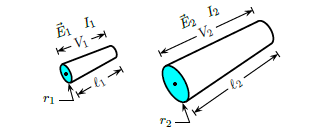Problem: Consider two conductors 1 and 2 made of the same ohmic material (ρ 1 = ρ2). Denote the length of the conductor by ℓ and the radius by r. The same voltage V is applied across the ends of both conductors. If r2 = 2 r1 , and ℓ2 = 2 ℓ1 , find the ratio I 2 / I1 of the currents. 1. I2 / I1 = 8 2. I2 / I1 = 1/2 3. I2 / I1 = 1 4. I2 / I1 = 1/4 5. I2 / I1 = 1/8 6. I2 / I1 = 2 7. I2 / I1 = 4

85% (66 ratings)
Problem Details

Consider two conductors 1 and 2 made of the same ohmic material (ρ 1 = ρ2). Denote the length of the conductor by ℓ and the radius by r. The same voltage V is applied across the ends of both conductors. If r2 = 2 r1 , and ℓ2 = 2 ℓ1 , find the ratio I 2 / I1 of the currents.

1. I/ I1 = 8

2. I/ I1 = 1/2

3. I/ I1 = 1

4. I/ I1 = 1/4

5. I/ I1 = 1/8

6. I/ I1 = 2

7. I/ I1 = 4